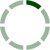### Posts by: Yong Heng T

How does non-controlling interest affect the income statement model & calculation of EPS?
Hi, I understand that EPS is the net income attributed to ordinary shareholders divided by the weighted average number of ordinary shares. So EPS = (Net Income - Non-controlling interest) / Weighted average number of ordinary shares? Do we also deduct the non-controlling interest from the Operati... Read More
Go to post added 6 years ago